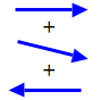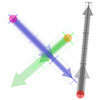You may also likeArrow Arithmetic 1

The first part of an investigation into how to represent numbers using geometric transformations that ultimately leads us to discover numbers not on the number line.Arrow Arithmetic 2

Introduces the idea of a twizzle to represent number and asks how one can use this representation to add and subtract geometrically.Arrow Arithmetic 3

How can you use twizzles to multiply and divide?

Twizzle Wind Up

Age 14 to 16 Challenge Level:

We're going to start with the same animation we looked at in Twizzles Venture Forth . If you haven't done so yet, it would be worth getting in some twizzle arithmetic practice using the notes for that problem as a guide.

In this animation, you can change the value of the blue twizzle z .

The red twizzle takes the value (z-i) .

The green twizzle takes the value (z+i) .

The grey twizzle takes the value (z-i)(z+i) .

Full Screen Version

If you can see this message Flash may not be working in your browser
Please see http://nrich.maths.org/techhelp/#flash to enable it.
The equation (z-i)(z+i) = 0 has two solutions; z=i and z=-i .

Switch on 'Show Tracings' and draw a small loop around each of these solutions with the blue z twizzle. Observe carefully what happens to the paths traced by the red, green, and grey twizzles and how the paths relate to each twizzle's zero spot.

Now click on 'Show Tracings' twice - once to clear the paths, and once to switch tracing back on, and draw a small loop around the other solution. Again, observe carefully the paths of the other twizzles.

Click 'Show Tracings' twice again to clear the paths.
This time, draw a loop that goes around both solutions (so both the solutions are inside your loop). Again, observe what paths the other twizzles trace out. How many times to they turn around their spots?

Here's the question!

When you trace out a loop with the blue z twizzle, what determines how many times the grey (z-i)(z+i) twizzle winds around its zero spot?

Generalise!

You might like to experiment some more with the next slightly more general animation. You can change the value of the beige a twizzle as well as the value of the blue z twizzle. Roll over the twizzles to see how they are calculated.

Something special happens when the beige a twizzle is zero. What is it?:

Full Screen Version :
If you can see this message Flash may not be working in your browser
Please see http://nrich.maths.org/techhelp/#flash to enable it.